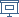FLOC 2018: FEDERATED LOGIC CONFERENCE 2018
PC ON SUNDAY, JULY 8TH
Days:
previous day
all days

View: session overviewtalk overviewside by side with other conferences

09:00-10:30 Session 34L: Joint QBF / Proof Complexity session (joint with QBF)
Location: Maths L5
09:00
Lower Bound Techniques for QBF Proof Systems
10:00

ABSTRACT. Towards the Semantics of QBF Clauses

10:30-11:00Coffee Break
11:00-12:30 Session 38N: Joint QBF / Proof Complexity session (joint with QBF)
Location: Maths L5
11:00
Size, Cost and Capacity: A Semantic Technique for Hard Random QBFs
11:30
Short Proofs in QBF Expansion
11:50
The Symmetry Rule for Quantified Boolean Formulas
SPEAKER: Martina Seidl
12:10
Discussion
12:30-14:00Lunch Break
14:30-15:30 Session 41
Location: Maths L6
14:30
Complexity of expander-based reasoning and the power of monotone proofsABSTRACT. One of the main successes in circuit complexity is the strong lower bounds on complexity of monotone circuits. By analogy, one might expect that studying monotone reasoning would lead to similar lower bounds in proof complexity. Yet surprisingly, Atserias, Galesi and Pudlak have given a general quasipolynomial simulation of sequent calculus LK by its monotone fragment MLK. Moreover, their techniques give a polynomial simulation, provided properties of AKS sorting networks can be formalized inside LK. Such formalization was obtained in 2011 by Jerabek, assuming provable in LK existence of expander graphs.

Several major results in complexity theory such as undirected graph reachability in logspace (Reingold, Rozenman-Vadhan) and monotone formulas for sorting (Ajtai-Komlos-Szemeredi sorting networks) are based on properties of expander graphs. But what is the complexity such proofs? Much of the existing expander constructions rely on computationally non-trivial algebraic concepts for the analysis, such as the spectral gap, even when constructions themselves are combinatorial.

In this work, we show that existence of expanders of arbitrary size can be proven using NC^1 reasoning. We give a fully combinatorial analysis of an iterative construction of expanders using replacement product, powering and tensoring, and formalize this analysis in the bounded arithmetic system VNC^1. Combined with Atserias, Galesi, Pudlak'2002 and Jerabek'2011, this completes the proof that monotone LK is as powerful as LK for proving monotone sequents.

Joint work with Sam Buss, Valentine Kabanets and Michal Koucky.

15:00
Towards theories for positive polynomial time and monotone proofs with extension

ABSTRACT. We propose arithmetic theories that link systems in monotone proof complexity to classes in monotone computational complexity. In particular, we focus on the case of polynomial-time, for which monotone function classes and monotone proof systems have recently been proposed. We complete the proof complexity theoretic account of this subject by proposing an accompanying logical theory, in the usual sense of 'bounded arithmetic'

15:30-16:00Coffee Break
16:00-17:50 Session 42N
Location: Maths L6
16:00
A Comparison of Algebraic and Semi-Algebraic Proof Systems
17:00
Optimization over the Boolean Hypercube via Sums of Nonnegative Circuit Polynomials
SPEAKER: Adam Kurpisz

ABSTRACT. Various key problems from theoretical computer science can be expressed as polynomial optimization problems over the boolean hypercube. One particularly successful way to prove complexity bounds for these types of problems are based on sums of squares (SOS) as nonnegativity certificates. In this article, we initiate optimization problems over the boolean hypercube via a recent, alternative certificate called sums of nonnegative circuit polynomials (SONC). We show that key results for SOS based certificates remain valid: First, for polynomials, which are nonnegative over the \$n\$-variate boolean hypercube with constraints of degree \$d\$ there exists a SONC certificate of degree at most \$n+d\$. Second, if there exists a degree \$d\$ SONC certificate for nonnegativity of a polynomial over the boolean hypercube, then there also exists a short degree \$d\$ SONC certificate, that includes at most \$n^{O(d)}\$ nonnegative circuit polynomials. Finally, we show certain differences between SOS and SONC cones namely, we prove, that in opposite to SOS, SONC cone is not closed under taking affine transformation of variables and that for SONC there does not exist an equivalent to Putinar's Positivestellensatz for SOS. We discuss these results both from algebraic and optimization perspective.

17:30

ABSTRACT. This work overviews recent results on the dual-rail based MaxSAT solving, including polynomial upper bounds on the refutation of PHP and 2PHP formulae with core-guided MaxSAT solvers and MaxSAT resolution as well as their relative efficiency compared to general resolution and cutting planes.